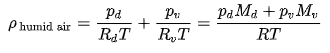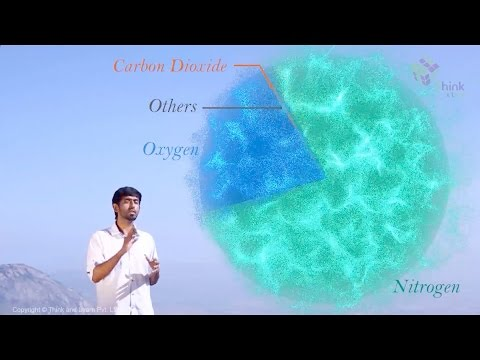# Density of Air

Density is a measure of mass. The formula for density is d = m/v, where d is the density, m is the mass, and v is the volume. In this session, let us discuss the density of air, value of air density, and factors affecting the density of air.

## What is Air Density?

Air density is denoted by the Greek letter rho,

$$\begin{array}{l}\rho\end{array}$$
. The value of the air density given is at STP.

An object made from a comparatively dense material will have less volume than an object of equal mass made from some less dense substance. The highest density known is of a neutron star. A brick and a loaf of bread are about the same size, but a brick is heavier–it is denser. Among metals, aluminium is less dense than iron. That’s why aeroplanes and some automobile parts are made from aluminium. For the same volume of material, one metal weighs less than another. The density of a pure gold nugget on earth and moon would measure the same. But for gases, because the molecules are free to move, the density can vary over a wide range. The air density at the surface of the Earth would have a different density than air 50 kilometres above the earth.

## What is The Value of Air Density?

The value of air density is 1.29 grams per litre (0.07967 pounds per cubic foot) at 32 degrees Fahrenheit (0 degrees Celsius) at average sea-level barometric pressure (29.92 inches of mercury or 760 millimetres).

### Density of Dry Air

The density of dry air is calculated using the ideal gas law as follows,

$$\begin{array}{l}\rho =\frac{p}{R_{specific}T}\end{array}$$

where:

$$\begin{array}{l}\rho\end{array}$$
is the air density (kg/m3)

p is the absolute pressure (Pa)

T is the absolute temperature (K)

Rspecific is the specific gas constant for dry air (J/(kg.K))

### Density of Humid Air

The density of the air reduces due to the addition of water. This is because the molar mass of water is less than the molar mass of dry air. For an ideal gas at a given temperature and pressure, the number of molecules is constant for a given volume of air. The density of humid air is calculated as follows:where:

Phumid is the density of the humid air

Pd is the partial pressure of dry air

Rd is the specific gas constant of dry air

T is the temperature

pv is the pressure of water vapour

Rv is the specific gas constant for water vapour

Md is the molar mass of dry air

Mv is the molar mass of water vapour

R is the universal gas constant

## Factors Affecting the Density of Air

• Air becomes denser as the air pressure increases. The pressure forces the air molecules together resulting in more mass in a given volume. Increasing altitude results in decreasing air pressure. The decrease in air density means that a mountain climber at high altitudes gets less oxygen when he breathes.
• Temperature is another factor that affects the density of air. When the temperature is increased, the air molecules move faster and spread further apart on collision. When air is denser, it creates a drag on objects moving through it. For example, a golf ball hit on a hot summer day will go further apart than one hit on a cold day. The higher temperature and lower air pressure found at high altitudes combine to lower the air density.
• Humidity or the amount of moisture in the atmosphere also changes the density of air. More moisture in the air, lower the density of air.

Watch the video below to learn about the composition of air and its properties in detail.## Frequently Asked Questions on Density of Air

### Which instrument is used to measure the air pressure?

Barometer is used to measure air pressure.

### What causes wind?

Wind is caused by differences in atmospheric pressure. When a difference in atmospheric pressure exists, air moves from the higher to the lower pressure area, resulting in winds of various speeds blowing in different directions.

### Where is the air density the highest?

The density of the air is highest near the ground (troposphere) and gets less and less as you rise in altitude.

### What is density and how is it measured?

Density is a measure of mass. The formula for density is d = m/v, where d is the density, m is the mass, and v is the volume.

### State true or false: The density of the air increases due to the addition of water.

False. The density of the air reduces due to the addition of water.

Test your knowledge on Density of air